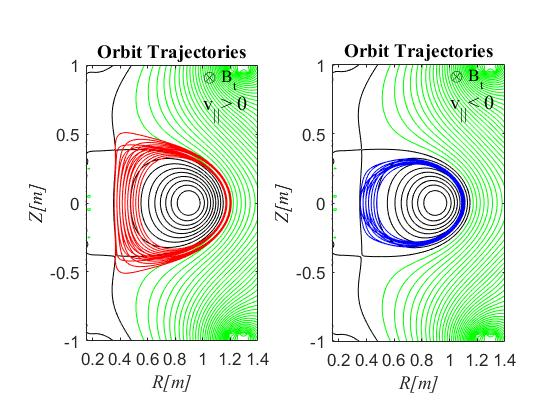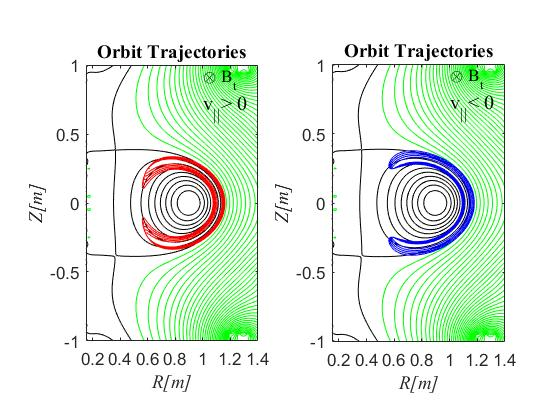Since 18 of December 2019 conferences.iaea.org uses Nucleus credentials. Visit our help pages for information on how to Register and Sign-in using Nucleus.

# 28th IAEA Fusion Energy Conference (FEC 2020)

May 10 – 15, 2021
Virtual Event
Europe/Vienna timezone
The Conference will be held virtually from 10-15 May 2021

## Relativistic Electrons’ Orbit Trajectory Calculation and Calculation Study analysis in Electron Cyclotron Heating and Current Drive of Tokamak Plasmas

May 11, 2021, 2:00 PM
4h 45m
Virtual Event

#### Virtual Event

Regular Poster Magnetic Fusion Theory and Modelling

### Description

The electron cyclotron heating (ECH) is one of the intense methods of non-inductive plasma current drive (CD). The ECH waves accelerated the electrons with the Doppler-shifted electron cyclotron resonance (ECR) interactions, and effectively ramped and sustained the plasma current non-inductively to achieve long discharge duration. The plasma sustainment with ECH waves is a key issue for the steady state operation in the tokamak configuration. The plasma was sustained for more than 2 hours by the ECH/CD non-inductive method in the Q-shu University experiment with steady-state spherical tokamak (QUEST) 1. The plasma current generation as well as sustainment of the plasma is strongly related to the ECR conditions and confinements of the electrons in the magnetic field. In this study, the relativistic electrons’ orbit trajectories were calculated and analyzed under equilibrium magnetic configuration of spherical tokamak in the ECH/CD to evaluate the ECR conditions and confinements of the electrons in the magnetic field. A framework with several sets of calculation codes accordingly for various theoretical models was developed with the EFIT (Equilibrium Fitting) plasma equilibrium to calculate and analyses orbit trajectories.

This study observed that a large number of passing resonant electrons with initial positive $v_\parallel$ starting from the high field side were maintained their orbits outside the last closed flux surface (LCFS), while all the passing resonant electrons with initial negative $v_\parallel$ starting from the high field side were maintained their orbits inside the LCFS. The trapped resonant electrons only being the 2nd harmonic ECR were maintained banana orbits at the low field side of the torus where most of the portions of the banana orbits of initial positive $v_\parallel$ were placed outside the LCFS.

In this study, the equilibrium magnetic configuration was obtained from the plasma equilibrium solution using 129x129 (129 grid points in the R direction and 129 grid points in the $Z$ direction) spatial resolutions EFIT code. The poloidal flux $\psi$ and toroidal current density of plasma $J_T$ were calculated by the EFIT code on the rectangular grid with the external magnetics for constraint of a discharge that satisfy the model provided by the Grad-Shafranov equation 2.

The orbit trajectories of the resonant electrons were calculated and analyzed for the 8.2 GHz electron cyclotron heating and current drive waves on the equilibrium magnetic configuration of the Q-shu University experiment with steady-state spherical tokamak (QUEST) in the relativistic Doppler-shifted ECR. The down-shifted and up-shifted fundamental and second (2nd) harmonic relativistic resonances were considered separately. Various parallel and perpendicular velocities to the magnetic field, $v_\parallel$ and $v_\perp$ were considered from the Doppler-shifted ECR condition for the electrons to be resonant with the ECH waves. The parallel refractive index $N_\parallel$ from -1 to +1 with step 0.1 was taken into account in the multiple-wall reflection model. The maximum energy of the resonant electrons was restricted at 100 keV and calculated the orbits of those electrons whose initial energies were between 1 to 100 keV. The orbit trajectories of the ECR electron were obtained as contour plot of the resonant electron’s energy under the equilibrium magnetic configuration on the poloidal cross-section. The energy was expressed in terms of magnetic moment and toroidal angular momentum. The energy, magnetic moment, and toroidal angular momentum were conserved in the orbit trajectories. All the resonant and confined electrons’ orbits trajectories were obtained for various positions of the coordinates $(R, Z)$, resonant pitch angles and parallel refractive index. The number of the step parameters was more than 5,200000.

The red and blue contours in figure 1 show the calculated orbit trajectories on the poloidal cross-section between the $R$ positions from 0.35 m to 0.53 m and $Z$=0.00 m of the fundamental resonant electrons. The black and green contours show the closed and opened magnetic surfaces, respectively. The numbers of actually calculated orbit trajectories were much more than the orbits shown in the figure. The left and right figures show the orbit trajectories of the electrons with initial + $v_\parallel$ and - $v_\parallel$, respectively. Figure 2 shows the banana orbits of the trapped electrons on the poloidal cross-section of the 2nd harmonic resonant electrons. The number of actually calculated orbits was much larger than the orbits shown in the figure. The left figure shows the banana orbits of the trapped electrons with initial + $v_\parallel$. The right figure shows the banana orbits of the trapped electrons with initial - $v_\parallel$.Depending on the ECR for various conditions, the resonant electrons were travelled both in the parallel and the antiparallel directions to the magnetic field of the tokamak. The resonant electrons with initial positive $v_\parallel$ were travelled in the parallel direction to the magnetic field, while the resonant electrons with initial negative $v_\parallel$ were travelled in the antiparallel direction to the magnetic field. These two types of resonant electrons with initial positive and negative $v_\parallel$ contribute current in opposite directions, respectively. A significant number of confined electrons with initial positive $v_\parallel$ were maintained portions of the orbits in the open magnetic surfaces that may cause to shift the plasma outward along the equatorial plane. In the summary, several codes required to calculate and evaluate the ECR as well as orbits have been developed with the EFIT code. Various criteria of the relativistic ECR conditions were properly taken into consideration for the orbit analysis. Additionally, the developed code could be applicable to analyze the $\alpha$-particle (alpha) orbits of the burning plasmas.

1. Hanada K., et al 2017 Nucl. Fusion 57 126061
2. Havlicek, J., et al 2007 WDS’07 Proceedings of Contributed Papers Part II— Physics of Plasmas and Ionized Media WDS’07 234-239